# Shedding Light on Motion Episode 5: Graphing Free Fall

In Episode 5 of the Shedding Light on Motion series, Graphing Free Fall, we continue looking at graphs but pay particular attention to how graphs help us to understand the motion of objects that are either falling straight down or which have been launched vertically upwards into the air.

The Shedding Light on Motion series is a visual treasure trove of demonstrations, animations, and explanations of all things motion! To an extent we’re all familiar with motion because we all move and we see movement everywhere, but a detailed knowledge of motion has allowed us to build the wonderful modern world that we live in.

The preview video below contains a 4.5-minute excerpt followed by a 1-minute trailer.The Episode 5 Question Sheet for Students:QS5 Graphing Free Fall.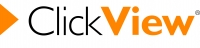If you have ClickView, watch the whole episode here.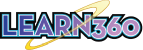If you have Learn360, watch the whole episode here.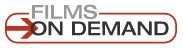If you have Films on Demand, watch the whole episode here.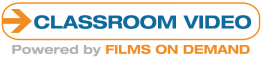If you have Classroom Video, watch the whole episode here.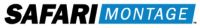Most of our videos are also available on SAFARI Montage. Just log in and do a quick search.Don’t have any of the above? Rent or buy the Shedding Light series and/or individual programs from our Vimeo page!!

The Transcript (which can be used as a textbook)

Graphing Free Fall Contents:
Part A: Falling Down. In the absence of air resistance, falling objects all display exactly the same behaviour when it comes to falling.
Part B: Launching Projectiles Upwards. What goes up must come down and graphs can elegantly show that the time it takes a projectile to reach the top of its flight is the same as the time it takes the projectile to fall back down again to its original height.
Part C: Curved Lines and Tangents. A tangent is a straight line that touches a curved line at a single point. The use of tangents is a really powerful method of analysing a graph that is curved.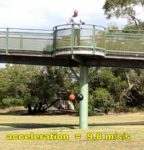Part A: Falling Down

We saw in our Acceleration program how objects in free fall, regardless of how heavy they are, accelerate at a rate of 9.8 m/s/s, at least initially before air resistance increases too much. In this episode of the Shedding Light on Motion series we’re going to graphically examine the motion of objects that are in free fall.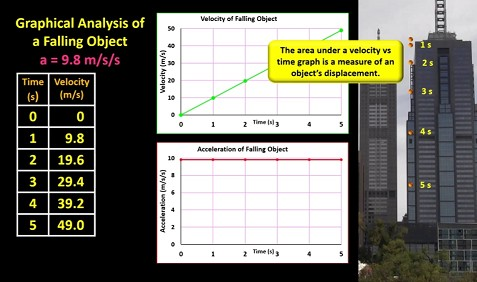Let’s look first at the motion of a falling ball dropped from a really tall building. Here we go. Drop! It’s not a real ball but the distances here are to scale. Since the acceleration of a falling object is 9.8 m/s/s, after falling for 1 second, an object will reach a velocity of 9.8 m/s downwards. Then, after 2 seconds, it will have gained another 9.8 m/s of velocity to reach 19.6 m/s, and then after 3 seconds it will have gained yet another 9.8 m/s of velocity to reach 29.4 m/s and so on. Drawing up a velocity vs time graph is fairly easy. There’s the first dot, there’s the second and so on. The velocity increases at a constant rate. The acceleration vs time graph is also fairly easy to draw. The acceleration is a constant 9.8 m/s/s downwards. That’s in fact why the velocity vs time graph has a constant gradient.
Now we saw in our last episode that the area under a velocity vs time graph is a measure of an object’s displacement so we can use this v-t graph to calculate the falling ball’s displacement after 1 second, 2 seconds and so on, and then graph the results.Let me get rid of the a-t graph first and put in a displacement vs time graph. At the beginning, the displacement was zero of course so our first dot goes here. By calculating the area under the velocity vs time graph up to the 1 second mark we can work out the ball’s displacement after 1 second. The area is half base times height which equals 4.9 metres. After one second the ball had fallen 4.9 metres so I can place a dot in this position.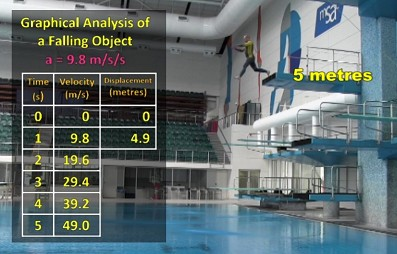In fact when I jumped off the 5-metre diving platform, I hit the water in 1.02 seconds, so our graphs so far fit with reality, which is always a good thing of course. After 2 seconds, the area under the v-t graph is half times 2 times 19.6 which equals 19.6 metres. This dot represents the information.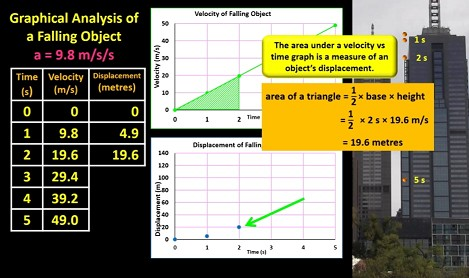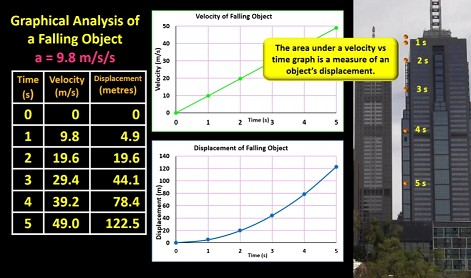You can see that as the ball got faster it covered more and more distance each second, so the displacement vs time graph curves upwards. Graphs can help us to really understand how objects move.
Looking at all three graphs at the same time, we can see that a constant acceleration leads to a steadily increasing velocity, which leads to an increasingly increasing displacement. We can use the graphs to work out lots of information.
For example: A ball is dropped from a tall building and it takes 4.2 seconds to reach the ground.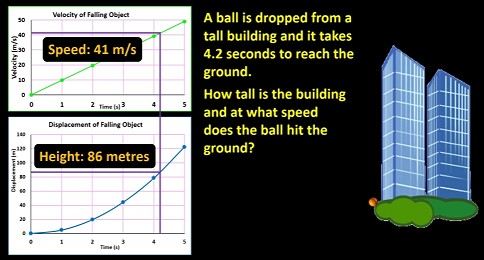How tall is the building and at what speed does the ball hit the ground? Reading off the v-t graph, we can see that the speed on impact was about 41 m/s. The displacement vs time graph shows that the ball must have fallen about 86 metres.
Let’s do another example. A ball is dropped from a 110-metre high cliff. How much time will it take to hit the ground, and at what speed will it hit the ground?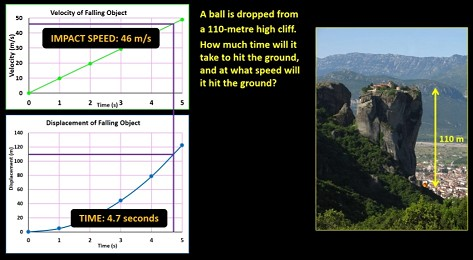Reading off the displacement vs time graph first, we can work out that the ball will take about 4.7 seconds. Using this information we can therefore work out from the velocity vs time graph that it will reach a speed of about 46 m/s (46.4 m/s). In both examples that we just looked at, I’ve assumed that air resistance is negligible.
Having answered these questions, might I suggest that if you do find yourself visiting a place that has tall cliffs, please resist the urge to start throwing things down to the ground below.

Part B: Throwing Up Launching Projectiles Upwards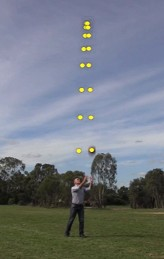Now what happens when you throw a ball upwards? When you throw a ball upwards, it slows down until it gets to the top of its flight and then it speeds up again as it falls back down. So how do we graph this motion? This one’s a little trickier, so before we graph it, it might be a good idea to go over some definitions again. Firstly we’ve seen that speed and velocity are very much related but velocity involves speed and direction.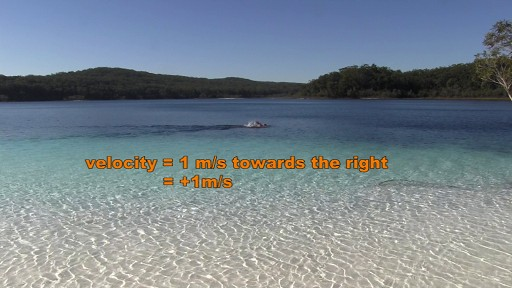Here I was swimming with a velocity of 1 m/s towards the right, or positive 1 m/s if we define the right as positive, while here I was swimming with a velocity of 1 m/s to the left or negative 1 m/s. In any given situation, you can define which direction you want to be positive. My speed in both clips here is the same, but my velocities are different.Distance and displacement are also related. Distance is how many, say, metres you actually travel, but displacement is how far away you are from your starting position and the direction that you’ve been displaced from your starting position.
When this ball, after having left my hands reached this point here, the distance it had moved was about 8 metres, but its displacement was about 1.5 metres up.
Acceleration also has a direction and we can say an object’s acceleration is towards the right or downwards, or negative, or whatever.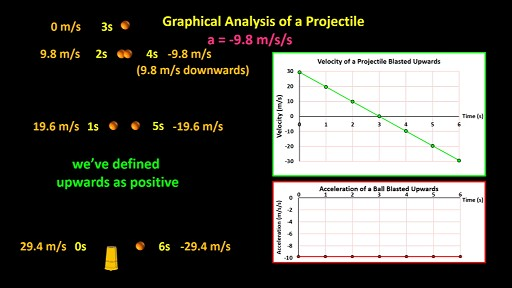So let’s examine the motion of a projectile which is blasted upwards with an initial velocity of 29.4 m/s. By initial velocity, I mean the velocity at which it left the cannon. We’ll start with a velocity vs time graph. We can draw a dot here to show that its initial velocity was 29.4 m/s upwards. We’ll define upwards as the positive direction in this example. We know its acceleration is -9.8 m/s/s, so we know that after 1 second it will have lost 9.8 m/s of its upwards velocity and so it will be travelling at only 19.6 m/s upwards. Let’s draw in the dot. After another second it slows down by another 9.8 m/s, so at the 2 second mark it’s moving at only 9.8 m/s upwards. Let’s mark it on the graph. One second later it loses another 9.8 m/s and so it ends up with a velocity of zero. Let’s put this dot in. What happens now? Well, we’ve just been subtracting 9.8 m/s every second so there’s no reason why we shouldn’t do exactly the same thing in the next second. 0 – 9.8 m/s = -9.8 m/s. After 4 seconds the projectile is moving with a velocity of -9.8 m/s, or in other words, 9.8 m/s downwards. On the graph, the dot goes here. Subtracting another 9.8 m/s/s gives us a velocity of negative 19.6 m/s, and finally after subtracting another 9.8 m/s, we end up with a velocity of -29.4 m/s as it reaches the same height that it started from. Joining the dots gives us a straight-line graph, which has a gradient of -9.8 m/s/s. (Now I should just point out that I’ve assumed that the projectile goes straight up and down, but I’ve drawn the path with a slight curve so that you can see the position of the projectile at the various stages that we’ve looked at.)
Let’s now fill in an acceleration vs time graph. The acceleration is negative 9.8 m/s/s the whole time so this graph is easy to draw. We simply draw in a horizontal line at the -9.8 m/s/s point. Now you might ask, how can the acceleration of the projectile be negative in the second half of its flight when it’s getting faster? Well, the acceleration is negative because in this example we’ve defined upwards as positive. A projectile accelerating downwards is increasing its velocity in the negative direction, so its acceleration is in the negative direction.
So, a negative acceleration can actually mean two things: either the object is slowing down, or it’s speeding up in the opposite direction to the direction that you define as positive. I hope that’s not too tricky.Let’s now use our v-t graph to draw up a displacement vs time graph. How far did the projectile travel in the first second after it was blasted upwards? Well, the shaded area of the v-t graph will give us that information. Now the shaded area to the 1-second mark is in the shape of a trapezium. Let’s do a quick Maths lesson. A trapezium is a four-sided shape that has 2 edges which are parallel to each other and two edges which are not parallel. The formula for calculating the area of a trapezium is A = half x (a+b) x h, where h is the distance between the two parallel lines. So, putting the numbers in we get A = half x (29.4 + 19.6) x 1 which equals 24.5 m. That’s how far the projectile moved in the first second.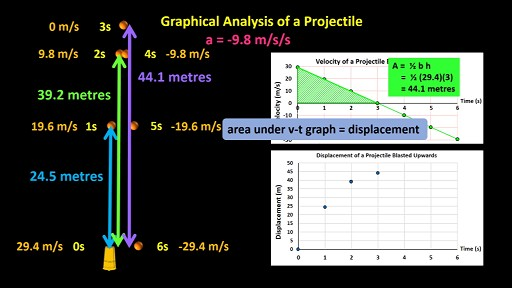At the beginning, the displacement was zero, so our first dot on the graph goes here, and at the one second mark, the displacement was 24.5 metres, so our next dot goes here.
The area up to the 2-second mark equals 39.2 metres. This is the height that the projectile reached after 2 seconds. Our next dot goes here. After 3 seconds, using the simple area of a triangle formula, A = half base x height, we get 44.1 metres. The projectile reached its maximum height of 44.1 metres after 3 seconds, and our next dot goes here.Now comes the tricky bit. Where was the ball after 4 seconds? As we’ve just seen, the area of this part of the graph is 44.1 metres. The area of this part of the graph though, is half base time height, which is half times 1 times negative 9.8 which is negative 4.9 m. Remember it’s not really area like you measure the area of a sports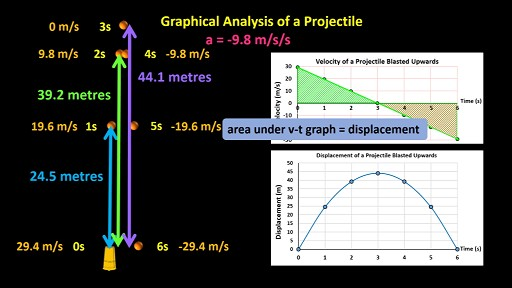ground. It’s the area in the dimensions of the graph, which, as I said, is -4.9 m. So, the total area up to the 4-second mark (between the line and the x-axis) is 44.1 + -4.9, which is 39.2 metres. The projectile was moving back to where it had been launched from and was only 39.2 metres away. As you can see it was at the same height that it had been two seconds earlier. The next dot on the displacement vs time graph goes here. If we do the same thing up to the five-second mark, we get 24.5 metres, (since 44.1 + -19.6 = 24.5 m) and then at the six-second mark, we get a displacement of zero metres (since 44.1 + -44.1 = 0 metres). The projectile was back to the same level it had started from. We can now join the dots on the graph with a line. Remember, all this assumes that the projectile moved straight up and down, but I’ve made it move slightly to the side just for clarity.
So, just repeating, the area under a v-t graph doesn’t tell you the distance the projectile has moved but rather the displacement of the projectile, that is, how far it is from its starting position.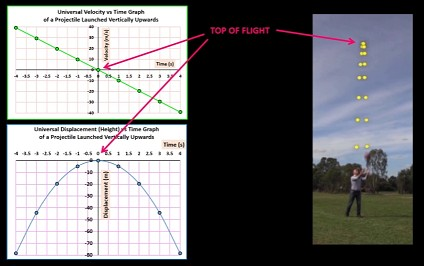This example of using graphs is a pretty tricky example, but hopefully you can now see just how powerful using graphs can be.
Since the upwards part of a projectile’s flight is the exact opposite of the downwards part of its flight, I can modify the displacement and velocity vs time graphs by assigning the top of the projectile’s flight as the zero second mark. The two graphs become kind of universal velocity and displacement vs time graphs and apply to any projectile launched upwards.
These sections are all about what happens before the projectile reaches the top of its flight, that is as it’s on its way upwards, and these sections gives us data about what happens after the projectile reaches the top of its flight, that is, as it descends.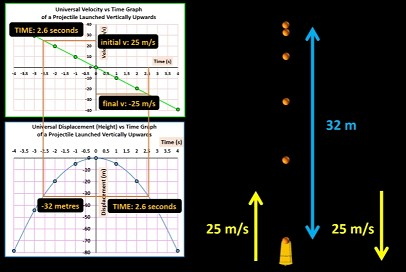So, for example, if a cannon ball is fired upwards with an initial velocity of 25 m/s, it will travel upwards for about 2.6 seconds before it reaches the top of its flight where it momentarily stops moving. The displacement vs time graph indicates that if it took 2.6 seconds to reach the top of its flight, then it must have been fired about 32 metres below the maximum height that it reached, which of course means it reached a height of 32 metres. It fell back down the same distance of course, 32 m, which according to the graph took 2.6 seconds. In fact, the upwards flight time will always be equal to the downwards flight time if the ball returns to its original level. After falling for 2.6 seconds, it will have reached a velocity of 25 m/s downwards, the same speed as the original speed but in the opposite direction.
I really like the way that the graphs so elegantly mirror what happens in real life.
The ball that I threw upwards here was in the air for exactly 1.96 (49 frames x 1/25 seconds per frame) seconds. For half of this time, 0.98 seconds, it was travelling upwards, and for the other half it was travelling downwards. Reading off the v-t graph, we can see that its initial velocity just as it left my hands must have been just under 10 m/s (actually 9.6 metres per second). Reading off the displacement vs time graph, when it left my hands, it was about 4½ metres below the maximum height that it reached, meaning it reached a height of about 4½ metres (actually 4.7 m) above the point that it left my hands. If I add my height, of about 1.8 metres, the ball reached a total height of about 6½ metres above the ground.

PART C: Curved Lines and Tangents

Let’s now look at one more advanced technique for analysing a motion graph.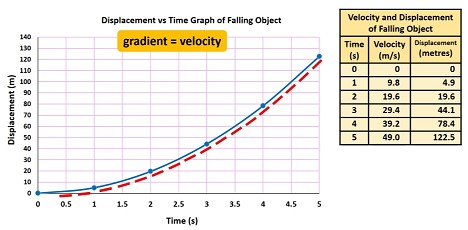This is a displacement vs time graph for an object in free fall. I said in our last episode, that on a displacement vs time graph the gradient of the line equals the velocity of the object, but how do you find the gradient of a curved line? A curved line doesn’t have a single gradient because its gradient keeps changing. However, we can calculate the gradient of the line at any given point that we choose. It helps if you can picture a curved line as series of straight lines that have different gradients.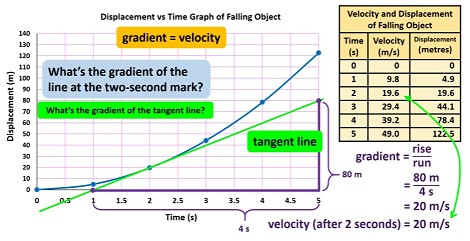For example, what’s the gradient of the line at the two-second mark? To work it out we need to draw what’s called a tangent line, or simply a tangent. A tangent is a straight line that touches a curved line at a single point and that points in the same direction as the curved line at that point.
The gradient of the tangent line here is the same as the gradient of the blue line on the graph at the 2-second mark. So what’s the gradient of the tangent line? Well we need to pick two points on the tangent and calculate the rise over the run. I always try, wherever possible, to pick two points that cross the grid lines, and then use these points to calculate the gradient, but of course that’s not always possible. The gradient in this case is the rise over the run which equals about 80 metres over 4 seconds (from the 1 second mark to the 5 second mark) which is about 20 m/s. So this is the velocity of the falling object after 2 seconds.
As we know, falling objects accelerate at 9.8 m/s/s, so after 2 seconds, they should reach 19.6 metres/second. So our calculation of the gradient is pretty accurate. Since it’s not always easy to draw a tangent line 100% accurately, or in fact even to read off a graph with 100% accuracy, in an exam, say, a little leeway is usually given.
The tangent of the curve at the three second mark looks something like this.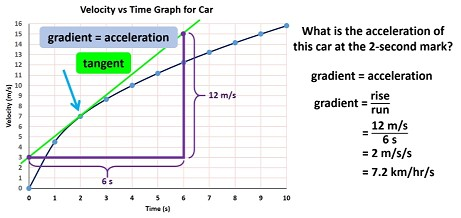Now we’ve seen previously that on a velocity vs time graph, the gradient equals the object’s acceleration. If the graph is curved, like it is here, but you want to know the acceleration of the object at any given moment, say the 2-second mark, well, once again, you have to draw a tangent to the graph’s line at the 2-second mark.
Remember a tangent touches a curved line at a single point, and points in the same direction as the curved line at that point.
So what is the acceleration of this car at the 2-second mark? Well, we choose two convenient points on the tangent, remembering though that the further apart the points are the more accurate your calculation is likely to be, and then use these points to find the gradient of the tangent which is the same as the gradient of the graph at the 2-second mark. The gradient and therefore the acceleration is a rise of 12 m/s over a run of 6 seconds, which is 2 m/s/s. This equates to 7.2 km/hr/s.To draw a tangent with a ruler, say at this point here, there’s a little bit of guess work involved, but this is too steep and this isn’t steep enough. You kind of zero in on the best angle and once you think you’ve got it right, just rule the line. That’s the tangent.
And that brings us to the end of our study of motion graphs. So far in this series we’ve looked at speed and velocity, acceleration, and relative motion, and we’ve analysed these things using equations and graphs. In our next episode we’re going to begin studying the forces that cause motion. See you then.

CREDITS:

Written and presented by Spiro Liacos

2016 Australian Gymnastics Championships – Day 11, Session 30 by ausgymnastics is used under a Creative Commons License.

2016 Australian Gymnastics Championships LARRISSA MILLER (VIC) Bars Event Final by ausgymnastics is used under a Creative Commons License.